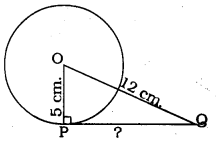# KSEEB SSLC Class 10 Maths Solutions Chapter 4 Circles Ex 4.1

KSEEB SSLC Class 10 Maths Solutions Chapter 4 Circles Ex 4.1 are part of KSEEB SSLC Class 10 Maths Solutions. Here we have given Karnataka SSLC Class 10 Maths Solutions Chapter 4 Circles Exercise 4.1.

## Karnataka SSLC Class 10 Maths Solutions Chapter 4 Circles Exercise 4.1

Question 1.
How many tangents can a circle have?
Solution:
There is only one tangent at a point of the circle.

Question 2.
Fill in the blanks :

1. A tangent to a circle intersects it in __________ point (s).
2. A line intersecting a circle in two points is called a ____________
3. A circle can have _____________ parallel tangents at the most
4. The common point of a tangent to a circle and the circle is called _____________ .

1. one
2. tangent
3. two
4. point of contact.

Question 3.
A tangent PQ at a point P of a circle of radius 5 cm meets a line through the centre O at a point Q so that OQ =12 cm, Length PQ is.:
a) 12 cm.
b) 13 cm.
c) 8.5 cm.
d) 19 cm.
Solution:
d) $$\sqrt{119} \mathrm{cm}$$.A tangent PQ at a point P of a circle.
OP is the radius drawn from centre of the circle to the tangent.
∴ OP ⊥ PQ.
Now, in ⊥ ∆OPQ, ∠P = 90°
∴ As per Pythagoras theorem,
OP2 + PQ2 = OQ2
(5)2 + PQ2 = (12)2
25 + PQ2 = 144
PQ2 = 144 – 25
PQ2 = 119
∴ √PQ2  = √119
∴ PQ = √119 cm.

Question 4.
Draw a circle and two lines parallel to a given line such that one is a tangent and the other, a secant to the circle.
Solution:CD is the tangent parallel to AB.
AB is secant, it meets RS at P.

We hope the given KSEEB SSLC Class 10 Maths Solutions Chapter 4 Circles Ex 4.1 will help you. If you have any query regarding Karnataka SSLC Class 10 Maths Solutions Chapter 4 Circles Exercise 4.1, drop a comment below and we will get back to you at the earliest.

error: Content is protected !!